Analysis of cell survival data

## Introduction

In this project I wanted to implement Root to analyze a datasheet provided to me from my suprevisors.

This datasheet contains information from radiation experiments where cells are irradiated by an

experimental radiation, in this case a proton beam, and a reference radiation, usually photons. We will

use Root to draw three scatterplots. One where the alpha value for the proton radiation is plotted to

the alpha value of the reference radiation. Another where the beta value of the proton radiation is

plotted to the beta value of the refenence radiation. And finally the LET-value plotted to the

RBEmin value.

## Theory

The linear quadratic (LQ) model is the most accepted model for cell survival studies. A central equation

of this model is the equation for survival fraction: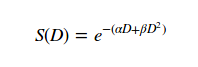Here S(D) is the fraction of the original cell population that survived the radiation and D is the dose per

But the two components we are interrested in from this equation are alpha and beta. Alpha is the linear

component of the LQ model. It contains information of the probablility for the particle to break both

the strains in the DNA of a cell. Beta is the quadratic component of the LQ model. It represent the

probability of two seperate single strain breaks in the DNA.

Another important concept is the Relative Biological Effactiveness (RBE) which is a measure of the

effectiveness of the experimental radiation (proton) compared to the reference radiation (photon). The

RBE is given by the equation: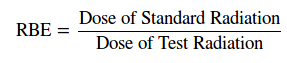But we are only interrested in RBE_min which is the RBE when only considering low dose. From 

can we see that RBE_min is given as :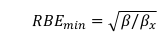Last we have the Linear Energy Transfer (LET). This describes the ionization density along the

particle trajectory. We are interrested in the Dose Averaged LET (LET_d). This is given as: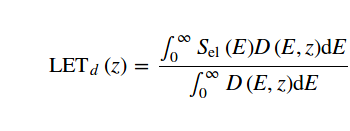In this equation S is the stopping power as a function of the beam energy and D is the dose deposited

in the depth z also as a function of the beam energy.

## Scripts

Tar file that contains everything I have used: Project

The textfiles was read into the codes and converted into .root files. Then each script created a plot.

Alphax_alphap.C plots a scatter plot where the alpha-value from the reference radiation is

x-coordinate and the alpha-value from the proton radiation is the y-coordinate. In the same

coordinate system the line x = y was drawn.

Betax_betap.C plots a scatter plot where the beta-value from the reference radiation is x-coordinate

and the beta-value from the proton radiation is the y-coordinate. In the same coordinate system the

line x = y was drawn.

LET_RBEmin.C plots a scatter plot where the RBEmin-value is x-coordinate and the LET-value

is the y-coordinate.

## Results

The codes provided the plots as shown in figures 1, 2 and 3.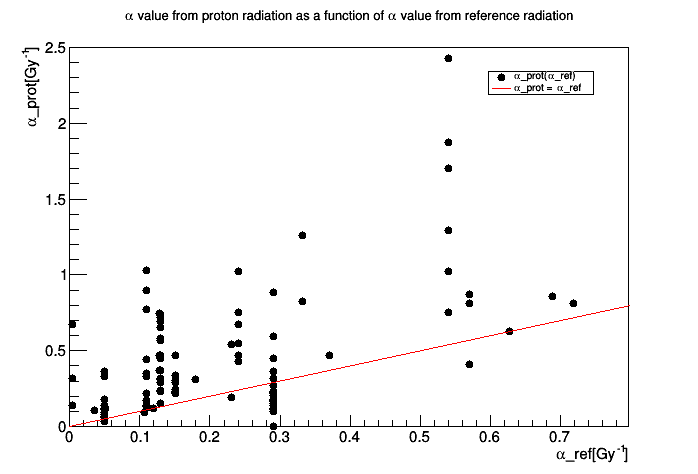Figure 1: Alpha from proton experiment plotted as a function of alpha from photon radiation.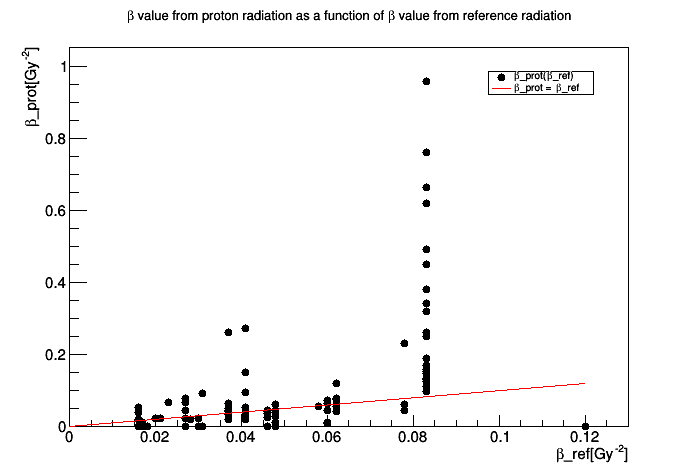Figure 2: Beta from proton experiment plotted as a function of beta from photon radiation.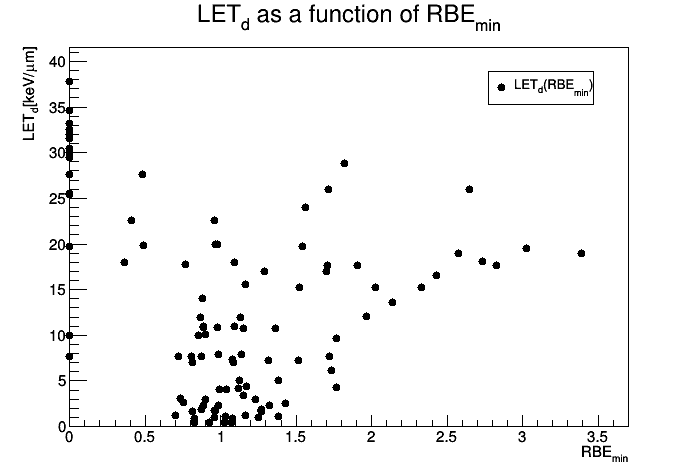Figure 3: Dose averaged LET plotted as a function of RBEmin.

## Conclusion/Discussion

From figure 1 we can see that most fo the alphas for the protons are higher for the ones for the photons.

This indicates that protons in most cases have a higher chance to make a double strain break, and

should therfore in theory be more effective at killing cells.

From figure 2 we see that especially for the low values, that the beta from proton and photon are much

more similar. This is not enough to say if either is more effective at killing cells by two single strain breaks.

Figure 3 shows that the data are very spread, especially for high LET. But for low LET the data is way

more gathered between the RBE values 0,5 and 1,8 and LET values up to about 8 kev/micrometer.

Finally I should mention that this is a verry basic analysis and there are many things that can be done in the

future. For instance to investigate the alpha/beta ratio.

## Scources and References:

 Rørvik. E, Thörnqvist. S, Ytre-Hauge. K. - The experimental dose ranges influence the LETd dependency of the proton minimum RBE (RBEmin)